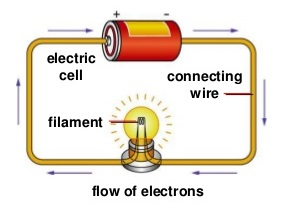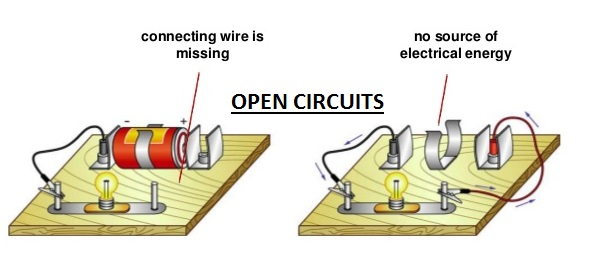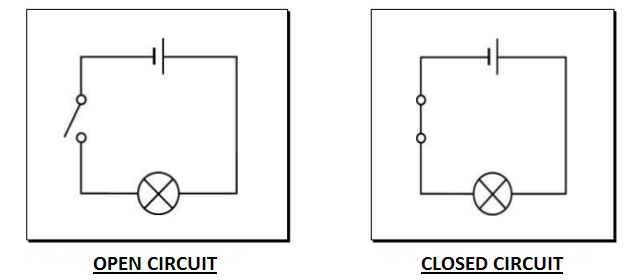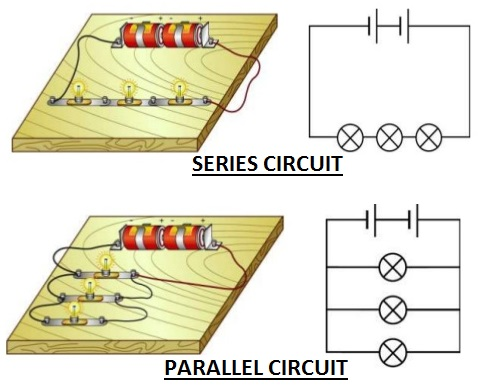# Define Electrical Energy & Electric Current

## What is Electricity?

Electricity is a form of energy that can be easily changed to other forms. Electricity comes mainly from 2 sources i.e.

1. Power stations and generators that supply a lot of electricity and normally used in many electrical appliances and industrial applications.
2. From Electric Cells or batteries which are used for portable purposes.

To make an electrical appliance work, electricity must flow through it. The flow of electricity is called an electric current. The path along which the electric current moves is called the electric circuit.

### What is an Electric Current?

1. An electric current is the rate of flow of electric charges in an electric circuit. Electric charges are made up of positive charges (protons) and negative charges (electrons). When these charges flow in a circuit, a current is produced.
2. The movement of electric charge is known as an electric current, the intensity of which is usually measured in amperes. Current can consist of any moving charged particles; most commonly these are electrons, but any charge in motion constitutes an electric current.How does electricity flow?

The battery in an electric circuit gives energy to the electrons and pushes them around a circuit, from the negative terminal of the cell, round the circuit and back to the positive terminal of the cell.

How to Measure Current?

The SI unit for electric current is ampere (A). Smaller currents are measured in milliamperes (mA).

1 ampere = 1 ??????? / 1 ??????

1 A = 1,000 mA

1 mA = 0.001 A

Different electrical components and appliances require different sizes of current to turn them on. An ammeter is an instrument used for measuring electric current. Electric currents are measured in amperes (A), hence the name. An ammeter should have a very low resistance so that it may not change the value of current flowing in the electric circuit.

### Electric Circuits

Electric circuits are connecting wires made up of electrical components, these components must be joined together without any gap in between to light bulb circuit board form a closed electric circuit. Incomplete circuits are called open circuits. An electric current flows only when there is:

• a source of electrical energy and
• a closed electric circuit connecting wiresBelow are typical circuit diagrams shown for open and closed electric circuits:#### Types of Electric Circuits

There are two types of circuits which are commonly used:

1. Series  Circuits
2. Parallel Circuits

Series Circuit: A series circuit connects the components one after the other. A single loop is formed. A break in any part of a series circuit stops the flow of current in the whole electric circuit.

Parallel Circuit: A parallel circuit divides into two or more branches. The current divides and flows through each parallel branch. If a component breaks or is removed, the other components remain on.Define Electrical energy

Electrical energy means work done in a period of time. Electrical energy is measured in Joules (in SI units). Since a watt is just a joule per second (J/s), a kilowatthour is a measure of energy which is normally used every where.

(1 kW hr = 1000 W hr = 1000 J/s hr = 1000 J/s x 3600s = 3.6 x 106 J)

Kilowatt Hours (kwh) = power x time = EMF x current x time

Electric Energy Explained:

H = I2 Rt gives the rate at which electric energy is dissipated or consumed in an electric circuit. This is also termed as electric power. The power P is given by P = VI Or P = I2R = V2/R

The SI unit of electric power is watt (W). It is the power consumedby a device that carries 1 A of current when operated at a potential difference of 1 V. Thus, 1 W = 1 volt × 1 ampere = 1 V A

The unit ‘watt’ is very small. Therefore, in actual practice we use a much larger unit called ‘kilowatt’. It is equal to 1000 watts. Since electrical energy is the product of power and time, the unit of electric energy is, therefore, watt hour (W h). One watt hour is the energy consumed when 1 watt of power is used for 1 hour. The commercial unit of electric energy is kilowatt hour (kW h), commonly known as ‘unit’.

as also mentioned above  1 kW h = 1000 watt × 3600 second = 3.6 × 106 watt second = 3.6 × 106 joule (J)

### Heating effect of electric current

When electricity passes through a high resistance wire like a nichrome wire, the wire or conductor becomes very hot and produces heat. This is called the heating effect of current.

Applications of heating effect of electric current

There are many practical uses of heating effect of current. Some of the most common are as follows:

1. An incandescent light bulb glows when the filament is heated by heating effect of current, so hot that it glows white with thermal radiation (also called blackbody radiation).
2. Electric stoves and other electric heaters usually work by heating effect of current.
3. Soldering irons and cartridge heaters are very often heated by heating effect of current.
4. Electric fuses rely on the fact that if enough current flows, enough heat will be generated to melt the fuse wire.
5. Electronic cigarettes usually work by heating effect of current, vaporizing propylene glycol and vegetable glycerin.
6. Thermistors and resistance thermometers are resistors whose resistance changes when the temperature changes. These are sometimes used in conjunction with heating effect of current(also called self-heating in this context): If a large current is running through the nonlinear resistor, the resistor’s temperature rises and therefore its resistance changes. Therefore, these components can be used in a circuit-protection role similar to fuses, or for feedback in electric circuits, or for many other purposes. In general, self-heating can turn a resistor into a nonlinear and hysteresis circuit element.